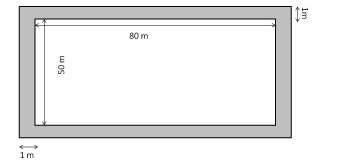# A rectangular ground 80 m × 50 m has a path 1 m wide outside around it.`
Question:

A rectangular ground 80 m × 50 m has a path 1 m wide outside around it. The area of the path is
(a) 264 m2
(b) 284 m2
(c) 400 m2
(d) 464 m2

Solution:

(a) 264 m2

Length of the ground including the path $=80+2=82 \mathrm{~m}$

Breadth of the ground including the path $=50+2=52 \mathrm{~m}$Total area (including the path) $=$ Length $\times$ Breadth $=82 \times 52=4264 \mathrm{~m}^{2}$

Area of the field $=80 \times 50=4000 \mathrm{~m}^{2}$

Area of the path $=4264-4000=264 \mathrm{~m}^{2}$